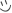# Decimals and fractions and rational and irational numbers

see how good you are at converting fractions to decimals and decimals to fractions. it is a very easy quiz for anyone doing 7-grade math it may be above please don't take if you are not above sixth or seventh grade it may be challenging

But no if you are below sixth or seventh grade and or are not in double advanced math please take this quiz if you want to be kinda prepared for seventh grade

Created by: Spitfire

1. what is 1/5 as a decimal
2. what's the equivalent of 4/5
3. what is 72/100 as a decimal
4. does -8/-10 =8/10
5. what is the equivalent of 12345/100000
6. what is 6/5 as a decimal
7. what is 188/11 as a mixed number
8. what is 44/11 as an integer
9. what is a rational can be a fraction
10. irrational numbers can be converted into fractions
11. irrational numbers can't be converted into fractions

You're about to get your result. Then try our new sharing options.